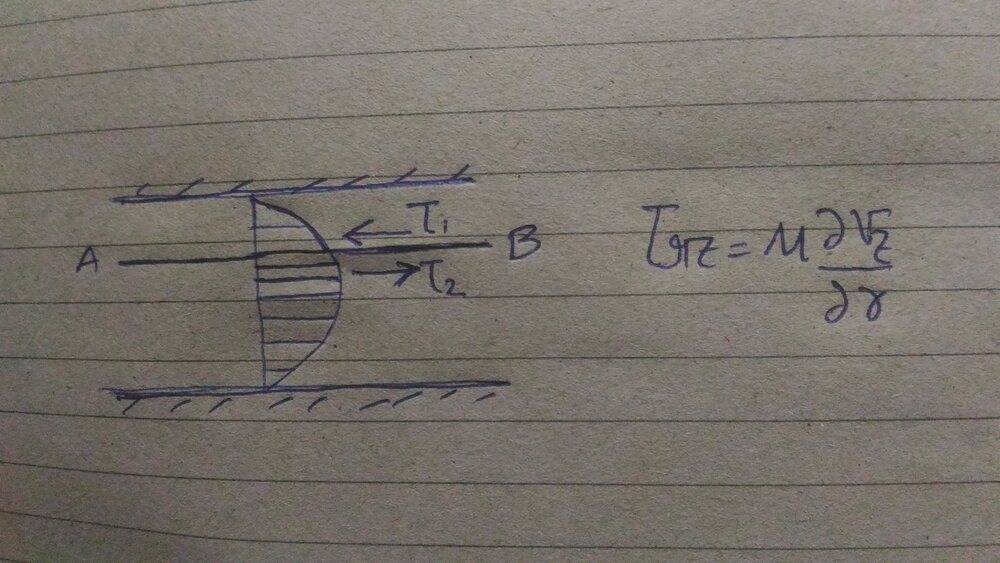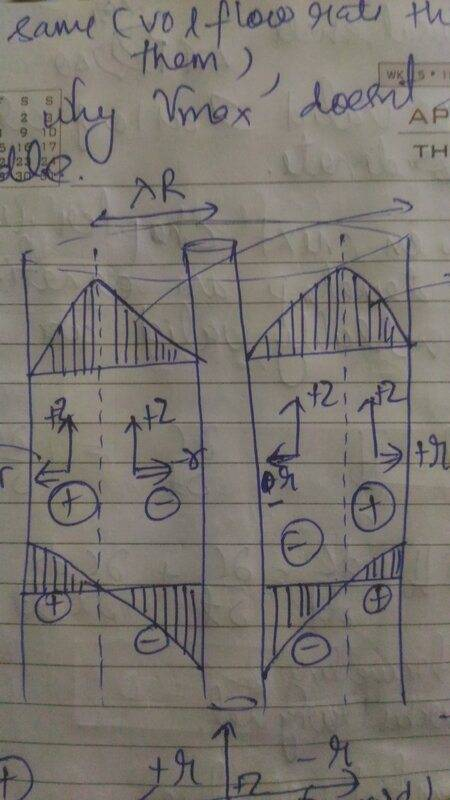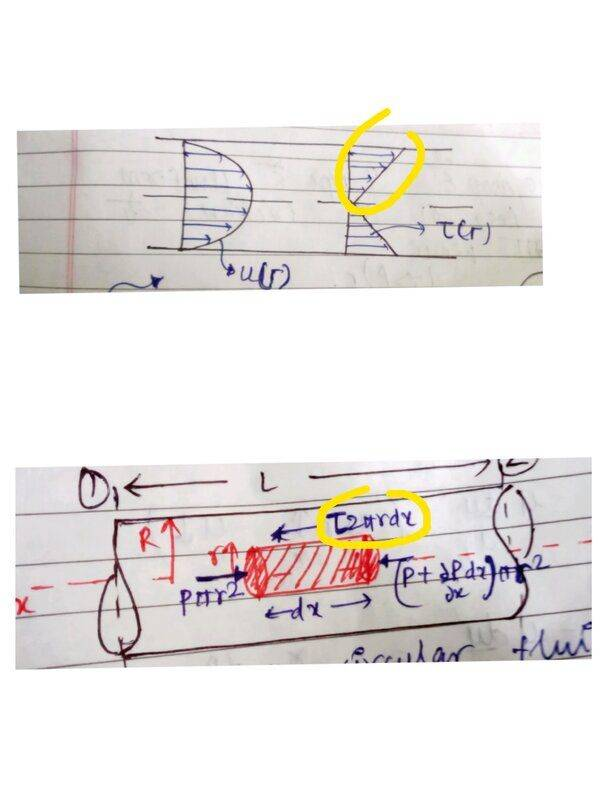# Some questions about Friction in fluid flow

Rahulx084
TL;DR Summary
Friction in fluid flow
I have few conceptual doubts in fluid mechanics. Here is the one I will ask first.

I want to understand the force direction acting on the solid liq interface during fluid flow, Let's say a fluid is flowing in a pipe. Now the fluid applies a shear stress at the walls of pipe in the direction of flow, from Newtons third law the wall will apply a backward force on the fluid which will be equal in magnitude to the shear stress times surface area. Is this reaction force applied by the wall is the same due to friction of surface of wall? I mean to say ,like when we push a solid block on a floor we have a friction force resisting the motion, In our fluid flow case , is the reaction force from wall same as the resistive force due to pipe wall friction, are they both same?

Gold Member
They are same. Newton's 3rd law applies for fluid and the wall.

Mentor
Simple answer is yes, friction against a surface is a force on the surface. And a force is a force.

Longer answer: Trying to make an analogy between fluid flow along a surface, and a block sliding on a surface, is not a good idea because the processes are different, which results in confusion. In both cases, there is a shear stress and an area. In both cases, the shear stress times the area is a force. In both cases, that force is applied to the surface.

In the fluid case, the shear stress is the result of a velocity gradient and viscosity. The fluid velocity at the surface is zero. The work to maintain the velocity gradient comes from a pressure difference in the direction of flow.

In the block case, the shear stress is the result of a force applied to the block. The block has a velocity relative to the surface on which it is sliding.

I suggest studying the first part of the Wikipedia article on viscosity. It's a good discussion and might help.

••ChemAir, russ_watters, FactChecker and 2 others
Homework Helper
Gold Member
... I want to understand the force direction acting on the solid liq interface during fluid flow, Let's say a fluid is flowing in a pipe... Is this reaction force applied by the wall is the same due to friction of surface of wall?...
The explanation about the Darcy–Weisbach equation could give you an idea of how complex the response to your excellent question can be.
https://en.m.wikipedia.org/wiki/Darcy–Weisbach_equation•berkeman
Mentor
The physical mechanism for viscous drag force on the fluid by the pipe wall is not the same as in dry friction. In the dry kinetic friction case, there is mechanical interference between the austerities in the wall surface and the the block surface. In the fluid flow case, the velocity of the fluid at the wall is zero. This is the so-called non-slip boundary condition. The zero velocity at the wall results in a velocity gradient that allows for momentum transfer between the fluid molecules at different distances from the wall, causing axial momentum to be transferred radially within the fluid. This is the mechanistic cause of the viscous wall drag.

••PhDeezNutz, Lord Jestocost, Rahulx084 and 1 other person
Rahulx084
Thanks everyone for the response, I need one confirmation . As Sir Chet said the mechanism of mechanical friction and fluid friction are different , when we study pressure drop in a pipe flow. We have friction factor term , which depends on pipe characteristics .Both friction factor and wall shear stress are related .

Friction factor= (Wall shear stress)/( Kinetic Energy Density)

The wall shear stress determined from above relation is the reaction force of the wall on the fluid and the action force is the stress on the pipe wall by fluid . Am I right? Also both are actually same .

Here is the second problem I wanted to ask,
In a laminar flow in pipe, we have a parabolic velocity profile. If we have to determine stress at a plane let's say AB which is at a location r, we will differentiate the velocity profile to determine the velocity gradient,then we will put it in Newton law of viscosity to determine stress at that point.
If we see the stress at above and below the plane , we see two stresses acting in opposite direction.
So is the stress obtained from Newton law via velocity gradient which was obtained from differentiation of velocity profile is equal to the net of the stress we have above and below the plane which in infinitesimal in thickness.Mentor
Thanks everyone for the response, I need one confirmation . As Sir Chet said the mechanism of mechanical friction and fluid friction are different , when we study pressure drop in a pipe flow. We have friction factor term , which depends on pipe characteristics .Both friction factor and wall shear stress are related .

Friction factor= (Wall shear stress)/( Kinetic Energy Density)

The wall shear stress determined from above relation is the reaction force of the wall on the fluid and the action force is the stress on the pipe wall by fluid . Am I right? Also both are actually same .

Here is the second problem I wanted to ask,
In a laminar flow in pipe, we have a parabolic velocity profile. If we have to determine stress at a plane let's say AB which is at a location r, we will differentiate the velocity profile to determine the velocity gradient,then we will put it in Newton law of viscosity to determine stress at that point.
If we see the stress at above and below the plane , we see two stresses acting in opposite direction.
So is the stress obtained from Newton law via velocity gradient which was obtained from differentiation of velocity profile is equal to the net of the stress we have above and below the plane which in infinitesimal in thickness.View attachment 265612
The shear stress components of the stress tensor are the same on both sides of the plane, and determined by Newton's law of viscosity. The traction vectors on the two sides of the plane constitute and action-reaction pair, and are equal in magnitude and opposite in direction. So, of course, the net force on the (massless) plane is zero.

••PhDeezNutz and Rahulx084
Rahulx084
Thanks sir, things are much clearer now.
Here is another one.
In a double pipe, talking about flow in the annulus region we get stress profile something like this. What does the negative and positive in stress profile means?Mentor
In the inner region, the fluid at ##r^-## is pulling backwards on the fluid at ##r^+##. In the outer region, the fluid at ##r^-## is pulling forwards on the fluid at ##r^+##.

Rahulx084
Hello Sir Chet , Sorry for being very late to this , it was due to some personal reasons in this pandemic.

Here I will continue further,
In this picture , the stress is acting backward as seen on the element, but stress profile says its positive . I'm a bit confused in understanding the meaning of stress profile, Can you please elaborate your last statement a bit more ,also in the same fashion as your last statement , please do the same for this stress profile.Mentor
There are two sign conventions that are used for the stress tensor, and, thus, for determining the stress profile. One is to treat tensile stresses as positive and compressive stresses as negative. The other is to treat compressive stresses as positive and tensile stresses as negative. The former is used most often, but, apparently, in the development you are working with, the latter is used (Transport Phenomena by Bird et al??). In the convention you are using, when applying the Cauchy stress relationship, you draw a unit normal from ##r^-## to ##r^+##, dot this with the stress tensor, and this gives you the stress vector (both normal- and shear stress) exerted by the fluid at ##r^-## on the fluid at ##r^+##. If you had used the other convention, when you dotted the unit normal with the stress tensor, it would have given you the stress vector exerted by the fluid at ##r^+## on the fluid at ##r^-##, and the shear stress would have been in the negative x direction. I hope this makes some kind of sense. If you are using Bird et al, it is important that you read the Appendix on dyadic tensor notation for 2nd order tensors (like the stress tensor), which can explain this in more detail. I hope that this makes some kind of sense to you.

Rahulx084
How by looking at a profile I can know which notation is used? like for the profile of annulus region flow in a double pipe I asked in post #8 of this thread , how would I know which notation is used?

Mentor
How by looking at a profile I can know which notation is used? like for the profile of annulus region flow in a double pipe I asked in post #8 of this thread , how would I know which notation is used?
I can't make out what is happening in post #8. If the shear stress has the same sign as the velocity gradient, then the first notation is being used. If the shear stress has the opposite sign of the velocity gradient, then the 2nd notation is being used. It looks like your book uses the 2nd notation (compressive stresses are positive).

Rahulx084
Okay.
I have to clarify one thing from the link you shared.
While working on a fluid element which have many faces, we will write the stress tensor for the whole fluid element right ? Which can contain up-to 9 elements and then we can just dot it with the area vector to determine traction vector of the face on which we want to know the stresses ,We do not determine stress tensor for each face of the fluid element, Right?

Also I want to ask from your reply post#7 , if the net force will be zero why we would have a stress at that plane then on a stress profile ?

Last edited:
Mentor
Okay.
I have to clarify one thing from the link you shared.
While working on a fluid element which have many faces, we will write the stress tensor for the whole fluid element right ? Which can contain up-to 9 elements and then we can just dot it with the area vector to determine traction vector of the face on which we want to know the stresses ,We do not determine stress tensor for each face of the fluid element, Right?
The stress tensor is a point function, and, if the fluid is deforming, the stress tensor at each face will be different.
Also I want to ask from your reply post#7 , if the net force will be zero why we would have a stress at that plane.then on a stress profile ?
This is an action-reaction pair. If you are doing a force balance on body A (that is in contact with body B), you only include the force exerted by B on A, not the force exerted by A on B. So if you are doing a force balance on the portion of the fluid below radial position r, you only include the external force exerted on it by the fluid at ##r=r^+##.

•Rahulx084
Rahulx084
I'm so lucky to have you as a mentor, you sir you are great. Thanks a lot , This is clear to me now . At least for now hehe : )